﻿ Physical Substantiation of Huygens Principle and the Reciprocity Theorem

### Physical Substantiation of Huygens Principle and the Reciprocity Theorem

F. F. MendeOPEN ACCESSPEER-REVIEWED

## Physical Substantiation of Huygens Principle and the Reciprocity Theorem

B.I. Verkin Institute for Low Temperature Physics and Engineering, NAS Ukraine, 47 Lenin Ave., Kharkov, Ukraine

### Abstract

The Huygens principle says, that each element of wave front can be examined as the center of the second disturbance, which generates second spherical waves, and the resulting light field at each point of space will be determined by the interference of these waves. This principle is the basic postulate of geometric optics; however, it does not reveal physical nature of this phenomenon. Are examined the laws of the self-induction of such reactive elements as capacity and inductance, which made possible to base the physical bases of Huygens's principle. It is shown that with the connection to the sources of direct current and voltage they have the effective resistance, which depends on the time. Is examined the parametric self-induction of the elements indicated and it is shown that in the regime of parametric self-induction the capacity and inductance can not only derive energy from the power sources, but also return it into the external circuits. The new method of determining the velocity of propagation of constant stresses and currents in the long lines is developed. This task cannot be solved, using standard wave equations for the long lines. The physical special features of the work of the receiving directional Yagi antennas are examined and it is shown that the reciprocity theorem for such antennas is not carried out.

12
Prev Next

### Cite this article:

• Mende, F. F.. "Physical Substantiation of Huygens Principle and the Reciprocity Theorem." American Journal of Electrical and Electronic Engineering 2.6 (2014): 165-170.
• Mende, F. F. (2014). Physical Substantiation of Huygens Principle and the Reciprocity Theorem. American Journal of Electrical and Electronic Engineering, 2(6), 165-170.
• Mende, F. F.. "Physical Substantiation of Huygens Principle and the Reciprocity Theorem." American Journal of Electrical and Electronic Engineering 2, no. 6 (2014): 165-170.

 Import into BibTeX Import into EndNote Import into RefMan Import into RefWorks

### 1. Introduction

Many specialists consider that the capacity and inductance are reactive elements and cannot take away energy in the alternating current circuits or voltage . In order to be convinced of the fact that this is not always carried out, it suffices to conduct simple experiment. If we connect capacitor to the electric brush of alternating current, to and then open it, then it is possible to reveal that the capacitor is charged. If we repeat this experiment repeatedly, then it is possible to establish that a voltage drop across the terminals of capacitor varies in the limits from the zero values, to the amplitude value of a potential difference in the network. This fact attests to the fact that the capacitor can take away energy from the electric brush, since the energy, accumulated in the capacitor, is determined by the equation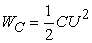where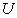is voltage drop across the terminals of capacitor.

To load capacitor is possible and from the source of direct current, and in this case the energy, accumulated in the capacitor, will be obtained from this source. Idea about the fact that the reactive elements cannot take away energy in the alternating current circuits, was formed for that reason, that with the examination of the properties of reactive elements in such chains are examined the periodic processes of infinite duration and the moment of turning off of reactive element from the chain is not considered.

If we charge capacitor from the source of direct current, then a voltage drop across it will be grow, and it will derive energy from the source, accumulating it similar to storage battery. In this case the capacity can be represented as the effective resistance, which depends on the time. The same relates also to the inductance, connected to the dc power supply. But capacity and inductance can return energy into the external circuit, if their value changes.

The special features of capacity and inductance indicated make it possible to solve a question about the velocity of propagation of constant voltage and currents in the long lines, what cannot be made, using telegraphic of equations.

Theory and different constructions of antennas are in detail examined in monographs [2, 3]. Primary attention in these works is given to the calculation of the transmitting antennas, at basis of which lies the principle of interference. However, up to now are not clear the physical the bases of the work of some types of the receiving directional antennas. With incomprehensibly what means the receiving Yagi antennas, which received very wide acceptance, ensure this high mu-factor. For guaranteeing the high mu-factor this antenna must gather energy from the lateral space, which surrounds antenna, but, as this it makes, the pill box of their times remains not clear.

### 2. Laws of the Self-induction

To the laws of the self-induction of reactive elements should be carried those laws, which describe the reaction of these elements with the galvanic connection to them of the sources of current or voltage. These laws are the basis of the theory of electrical chains. The results of this theory can be postponed also by the electrodynamics of material media, since such media can be represented in the form equivalent diagrams with the use of such elements .

By self-induction we will understand the reaction of material structures with the constant parameters to the connection to them of the sources of voltage or current. to the self-induction let us carry also that case, when its parameters can change with the presence of the connected power source or the energy accumulated in the system. This self-induction we will call parametric[4, 5, 6, 7].

Let us refine some terms, utilized in the article. We will call such generator, which ensures lumped voltage on any external circuit constant-potential generator. The internal resistance of the ideal voltage generator is equal to zero. We will call such generator, which provides the assigned current in any external circuit direct-current generator. The internal resistance of ideal current generator approaches infinity.

Ideal capacity cannot be connected to the ideal voltage source, since. at the moment of connection the current of the charge of capacity will approach infinity. Capacity can be connected only to the current source. Ideal inductance cannot be connected to the ideal current source, since. at the moment of connection a voltage drop across inductance will approach infinity. Inductance can be connected only to the dc power supply.

### 3. Capacitive Self-induction

If the capacity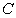is charged to a potential difference, then the charge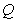, accumulated in it, is determined by the equationMagnitude of the charge can change with the method of changing the potential difference with a constant capacity, or with a change in the capacity with a constant potential difference.

If capacitance value or voltage drop across it depend on time, then the current strength is determined by the equation: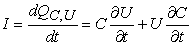Consequently, current in circuit can be obtained by two methods: changing voltage on the capacity with a constant capacity or changing capacity with constant voltage on it.

For the case, when the capacityis constant, we obtain(3.1)

Let us connect to the capacity direct-current generator and we will support in it the direct current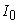. Then, after integrating Eq. (3.1) with respect to the time, we obtain(3.2)

This equation, which connects the direct current, which flows through the capacity, and voltage on it is the analog of Ohm law. For this reason the valueplays the role of effective resistance, which depends on time. The it should be noted that obtained result is completely obvious; however, such properties of capacity, which customary to assume by reactive element they were for the first time noted in the work .

The power, output by current source, is determined in this case by the equation:(3.3)

The energy, accumulated by capacity in the time, we will obtain, after integrating Eq. (3.3) with respect to the time: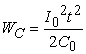Substituting here the value of current from Eq. (3.2), we obtain the dependence of the value of the accumulated in the capacity energy from the instantaneous value of voltage on it: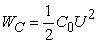If we support at the capacity constant voltage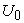, and to change capacity, then the current, which flows through it, is written down(3.4)

This case relates to the parametric self-induction. This equation, which connects the direct current, which flows through the capacity, and voltage on it is the analog of Ohm law. For this reason the value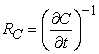plays the role of effective resistance, which depends on time.

The power, expended in this case by source, is determined by the equation: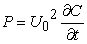From this equation is evident that depending on the sign of derivative the expendable power can have different signs. When the derived positive, expended by source power proceeds with an increase in the energy, stored up in the capacity. If derived negative, then work carries out the external source, which ensures the decrease of capacity. The energy, spent by this source, is separated in the external circuit.

### 4. Inductive Self-induction

Let us examine the processes, proceeding in the inductance. Let us introduce the concept of the flowVoltage on the inductance is equal to the derivative of the flow of current induction on the time: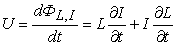Let us examine the case, when the inductance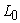is constant:(4.1)

Will maintaina constant voltage. Then, after integrating Eq. (4.1) with respect to the time, we obtain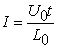(4.2)

This equation, which connects the direct current, which flows through the capacity, and voltage on it is the analog of Ohm law. Therefore the value ofplays the role of effective resistance, which depends on time.

The power, expended in this case by source, is determined by the equation: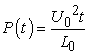(4.3)

After integrating Eq. (4.3) on the time, we will obtain the energy, accumulated in the inductance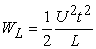After substituting in this equation the value of voltage from Eq. (4.2), we obtain the energy, stored up in the inductance,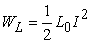If we support in the inductance the direct current, and to change inductance, then the current, which flows through it, will be written down(4.4)

This case relates to the parametric self-induction. This equation, which connects the direct current, which flows through the capacity, and voltage on it is the analog of Ohm law. Therefore the value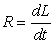plays the role of the effective resistance.

The power, expended in this case by source, is determined by the equation:As in the case with the capacity, effective resistance can be both the positive and negative. This means that the inductance can how derive energy from without, so also return it into the external circuits.

### 5. Propagations of Constant Voltage and Current in the Long Lines

The processes of the propagation of voltages and currents in the long lines determine the wave equations: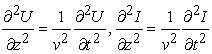which are obtained from the telegraphic equations: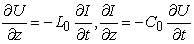whereand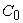are linear inductance and the capacity of line.

The velocity of propagation in this line is determined by the equation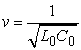The knowledge second derivative voltages and currents is required during the solution of wave equations.

But wave equations do not give the answer to the question, as one should enter when to incoming line it is connected dc power supply. The results, obtained in two previous divisions, give answer to these questions. The processes, examined in two previous paragraphs, concern chains with the lumped parameters, when the distribution of potential differences and currents in the elements examined can be considered uniform. However, there are chains, for example the long lines, in which the spatial distribution of voltages and currents they are not uniform.

Let us examine processes in the long line, whose capacity and inductance are the distributed parameters. We will consider that the linear capacity and the inductance of line is equalandrespectively. If we to this line connect the dc power supply o, then its front will be extended in the line some by the speed, and the moving coordinate of this front will be determined by the equation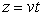. In this case the total quantity of the charged capacity and the value of the summary inductance, along which it flows current, calculated from the beginning lines to the location of the front of voltage, will change according to the law: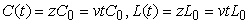The source of voltagewill in this case charge the being increased capacity of line, for which from the source to the charged line in accordance with Eq. (3.4) must leak the current: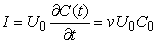This current there will be the leak through the conductors of line, that possess inductance. But, since the inductance of line in connection with the motion of the front of voltage, also increases, in accordance with Eq. (4.4), on it will be observed a voltage drop: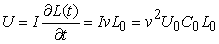(5.1)

But a voltage drop across the conductors of line in the absolute value is equal to the voltage, applied to its entrance; therefore in the last expression should be placed. Then from Eq. (5.1) we obtainThis equation coincides with the velocity of propagation, obtained from the wave equations.

However, in the electrodynamics, based on Maxwell equations, there are no such concepts as capacity and inductance, and there are concepts of the electrical and magnetic permeability of medium. In the carried out examination such concepts as electrical and magnetic fields also was absent. Let us show how to pass from such categories as linear inductance and capacity, current and voltage in the line to such concepts as dielectric and magnetic constant, and also electrical and magnetic field. For this let us examine the simplest construction of line, located in the vacuum (Figure 1)

Figure 1. The two-wire circuit, which consists of two ideally conducting planes

We will consider that>>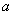and edge effects it is possible not to consider. Then the following connection will exist between the linear parameters of line and the magnetic and dielectric constants: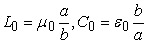whereand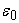is dielectric and magnetic constant of vacuum.

The velocity of propagation in this line is determined by the equationwhere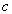is velocity of propagation of light in the vacuum.

The wave drag of the line examined will be equalwhere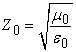is wave drag of free space.

This with the observance of the conditionwe obtain the equality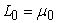. This means that magnetic permeability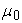plays the role of the longitudinal specific inductance of vacuum. In this case is observed also the equality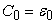. This means that the dielectric constantplays the role of the transverse specific capacity of vacuum. In this interpretation bothandacquire clear physical sense.

If we to the line of infinite length, connect the dc power supply, then the field strength in the line will be equal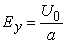and the current, which flows into the line from the power source, will be determined by the equation: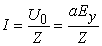Magnetic field in the line will be equal to the specific current, flowing in the lineSubstituting here the value of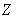, we obtain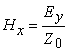The same connection between the electrical and magnetic field exists also for the case of the transverse electromagnetic waves, which are extended in the free space.

Comparing expressions for the energies, it is easy to see that the specific energy can be expressed through the electrical and magnetic fields(5.2)

This means that the specific energy, accumulated in the magnetic and electric field in this line is identical.

The special feature of this line will be the fact that in it, in contrast to the free space, the stationary magnetic and electric fields can be extended, but this case cannot be examined by the method of solution of Maxwell equations.

Consequently, conditionally it is possible to consider that the long line is the device, which with the connection to it of dc power supply is filled up with two forms of the energy: electrical and magnetic. The specific densities of these energies are equal, and since and electrical and magnetic energy fill identical volumes, the general energy, accumulated in these fields is identical. The distribution of electrical and magnetic pour on in the section from the beginning of line and to the front of voltage in it is uniform.

Is obvious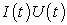represents the power, transferred through the cross section of line in the direction of. If in this equation current and voltage was replaced through the tensions of magnetic and electrical pour on, then we will obtain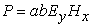. The work ofrepresents the absolute value of Poynting vector.

### 6. Physical substantiation of Huygens principle and the reciprocity theorem

Huygens principle says, that each element of wave front can be examined as the center of the second disturbance, which generates second spherical waves, and the resulting light field at each point of space will be determined by the interference of these waves. This principle is the basic postulate of geometric optics; however, it does not reveal physical nature of this phenomenon. From geometric optics it is known that any beam of light is dispersing and that the area of its section in the process of propagation always increases. This phenomenon is subordinated to Huygens principle. But is there any physical explanation of this principle? It occurs, there exists.

Let us examine flat monochromatic TEM the wave, passing through the slot, whose width is considerably longer more than wavelength (Figure 2).

After passage through the slot wave begins to be enlarged in the transverse direction, and this expansion is subordinated to Huygens principle. In this case in the expanding wave the ends of the paths of constant phase in the process of their motion move with the speed of light still, also, in the transverse direction. Difference is only the fact that in the line the wave of transverse electric field is propagated, and self-expansion occurs in the direction of propagation. In this case occurs the self-expansion of the vector of electric field still, also, in the transverse direction . In the long line there is no such expansion, since wave in the transverse direction I limit the conductors of line. The transverse transformation of wave is accompanied by the fact that, beginning from the center of path of constant phase along it begins leak bias current. This process is very similar to the expansion of the compressed elastic, when all its sections begin evenly to be enlarged. In this case the energy density of electromagnetic wave begins to decrease, being evenly distributed in the increasing volume, occupied by the expanding wave.

This simple examination, he indicates the physical causes for Huygens postulate and is in fact new physical law.

With this phenomenon is connected the so-called reciprocity theorem for the antenna systems, which does not up to now have its physical substantiation. This theorem says, that the mu-factor of the directional antennas is identical both with the emission and with the reception of signal. The strangeness of this theorem consists in the fact that the directional antenna can form the narrowly directed beam, when the radiated energy is concentrated in some direction. This means that the energy density is concentrated in the space limited from the lateral sides. This one can see well based on example of laser beam. However, receiving directional antenna is located in the fields of transmitter, which are evenly distributed in the space, and in order to increase its mu-factor to the directional receiving antenna it is necessary to know how to gather energy from the lateral space. This actually so, but as this it makes, until now, it remains riddle. A question does consist in that, is it possible to find some physical causes for this strange behavior of the directional receiving antennas.

Is known that the laws of geometric optics, when ray can be considered practically rectilinear, work when beam width considerably more than wavelength. In this case Huygens principle works. When the width of the slit becomes comparable with the wavelength, the slit is strongly divergent beam, and when the size of the gap becomes smaller the wavelength, the slit is radially divergent waves.

Let us examine two cases. In Figure 3 is depicted the case, when the width of obstacle is considerably lower than the wavelength.

Bend around obstacles such waves practically do not change their shape.

Figure 4. The case, when on the oscillation loop is located the obstacle, whose dimensions are greater wavelength

Quite different is the process of diffraction when the dimensions of the barrier longer wavelength (Figure 4).

Let us examine wave process in the long line, when on the way of propagation TEM wave is located the absorbing partition, whose dimensions are greater wavelength.

It is evident that from the right side from the absorbing obstacle there is a shadow zone, where the waves absent. But passing obstacle, the ends of the paths of constant phase begin to converge, bringing additional energy into the shadow zone from the lateral space. This process obeys the law of diffraction and has obvious special feature. Since the absorbing partition absorbed the part of the energy of the wave incident to it, the energy density of waves to the right beyond the limits of shadow, will be less than the energy density of waves to the partition. Direction the motions of energy in the expanding section of wave are shown by pointers. This means that in order to liquidate shadow and to restore normal wave process, wave energy begins to be pumped over from the lateral sections of those removed from the shadow zone, enlarging the sections of waves torn by obstacle. In this case Poynting vector to the right of shadow will be less than to the left of the partition. This means that as a result this diffraction process the specific energy again evenly was distributed on the perimeter of line, but its density will become less. If we on the route waves place consecutively several absorbing partitions of TEM wave, then after passage by the wave of sequential partition the Poyting vector will always decrease. For the realization of this process the wave energy always will be redistributed from the lateral regions line to the central region. This process is analogous to the process of the propagation of the wave front in the transmission line, only expansion of the lateral front of paths of constant phase occurs in the transverse direction and in the same direction is transferred the energy.

If the cross section of line is considerably greater than the section of the absorbing partitions, then a change in the vector of the Poyting vector will be insignificant, and each subsequent partition will absorb almost this energy as the previous partition. This connected with the fact that cross section fields on in the line considerably more than the cross section of partitions. But this process is connected with the fact that wave energy from the lateral sections, situated beyond the limits of the absorbing partitions, it is pumped over into the region of the arrangement of partitions.

If we instead of the absorbing partitions place the director of a Yagi antenna, then process will be repeated. But this will mean that this antenna uses not only wave energy, which directly falls to the directors, but also assembles energy from the surrounding space.

Let us make one refinement. We examined the case, when the directors of antenna were located at a distance, considerably larger than wavelength. But in a Yagi antenna the distance between the directors composes 0.25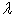. In this case such shadow, which is depicted on Figure 4., it cannot be formed after the directors. But, since each vibrator absorbs the specific share of energy of the wave incident to it, wave energy after the passage of this vibrator must decrease. But this it does not occur for that reason, that into the region of the arrangement of the following vibrator energy to be redistributed from the lateral regions. With this is connected the high mu-factor of the antenna examined.

However, to say that for the type of Yagiantennas performed reciprocity theorem, it is impossible, since the formation of directional transmitting antennabeam obeys the laws of interference, and the gain of the receiving antennais happening according to the laws of diffraction.

### Reference

  I. S. Gonorovsky. Radio Circuits and Signals. M.: Soviet Radio, 1977.In article  S. Ramo, John. Winner. Fields and Waves inmodernelectronics. OGIZ, 1948.In article  I. V. Goncharenko, Antennas HF and VHF. Part m5. Directional HF antenna. Shortened, phased, multi-band. Radiosoft, 2010.In article  F. F. Mende. Great errors and mistakes of physicists XIX-XX centuries. The revolution in modern physics. Kharkiv, NTMT 2010.In article  F. F. Mende New electrodynamics. Revolution in the modern physics. Kharkov, NTMT, 2012.In article  F. F. Mende, New approaches in contemporary classical electrodynamics. Part I, Engineering Physics, № 1, 2013.In article  F. F. Mende, New Properties of Reactive Elements and the Problem of Propagation of Electrical Signals in Long Lines, American Journal of Electrical and Electronic Engineering, vol. 2, no. 5 (2014): 141-145.In article CrossRef  F. F. Mende. Problems of modern physics and their solutions. Palmarium Academic Publishing, 2010.In article